Check Important Questions and Chapter Summary -https://you.tube/Real-Numbers-Class-10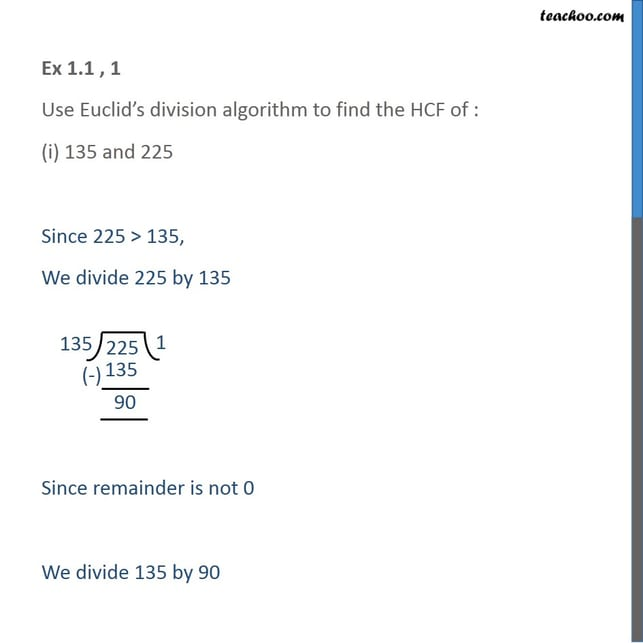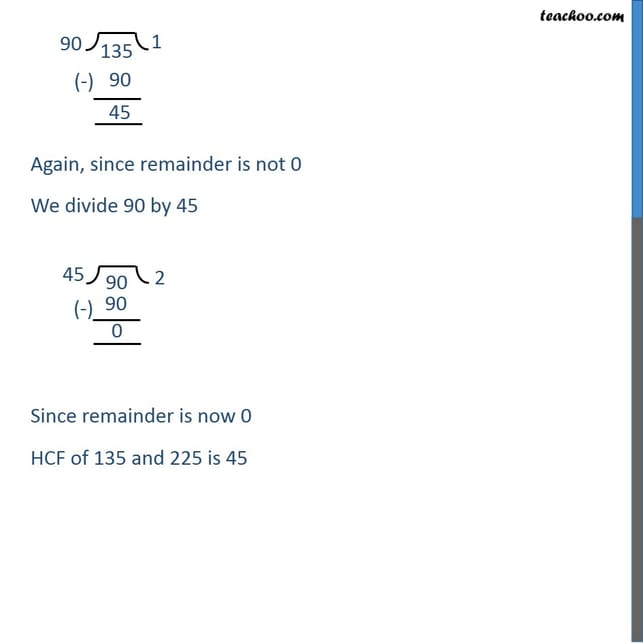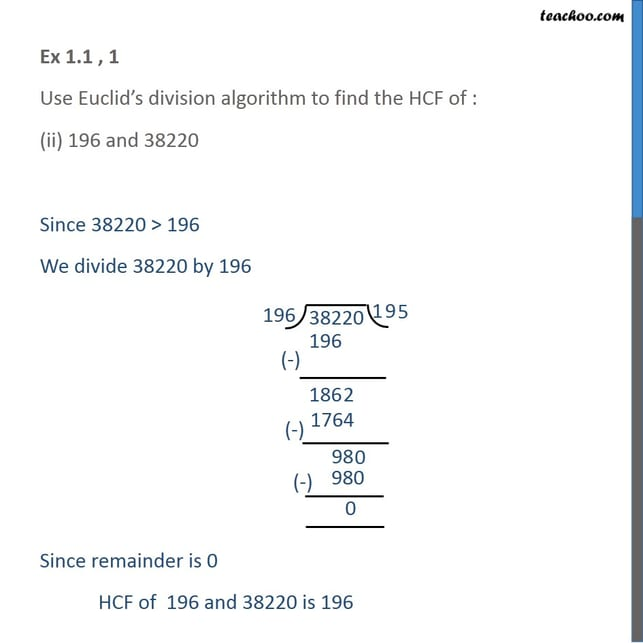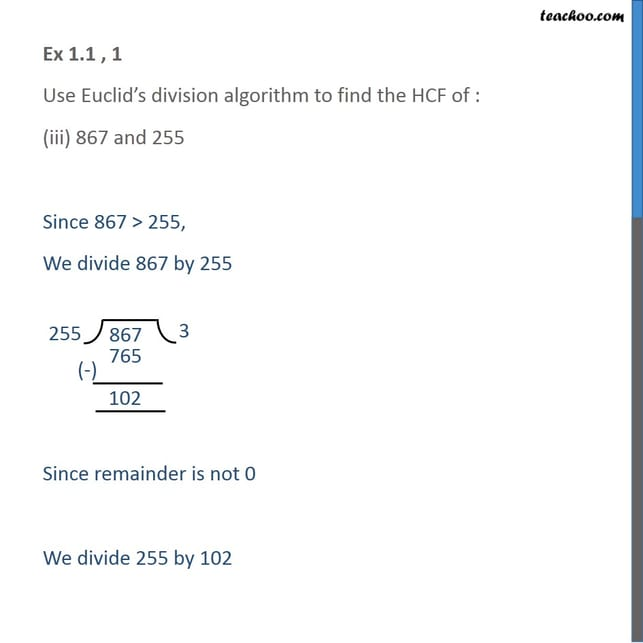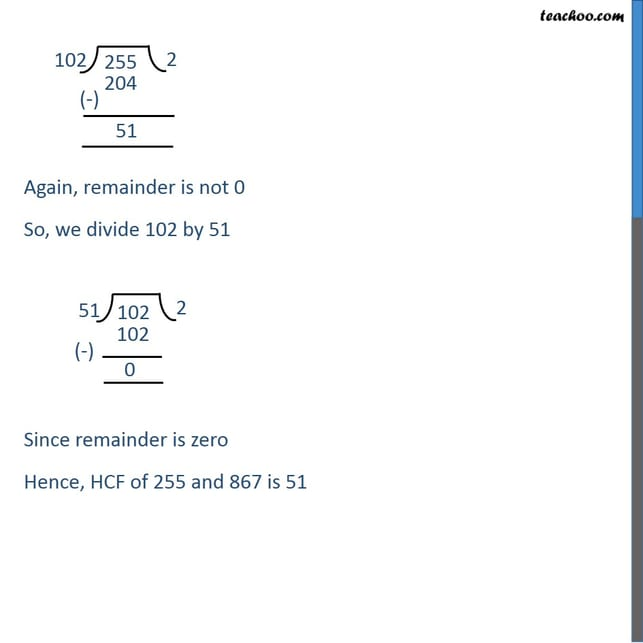1. Chapter 1 Class 10 Real Numbers
2. Serial order wise
3. Ex 1.1

Transcript

Ex 1.1 , 1 Use Euclid’s division algorithm to find the HCF of : (i) 135 and 225 Since 225 > 135, We divide 225 by 135 Since remainder is not 0 We divide 135 by 90 Again, since remainder is not 0 We divide 90 by 45 Since remainder is now 0 HCF of 135 and 225 is 45 Ex 1.1 , 1 Use Euclid’s division algorithm to find the HCF of : (ii) 196 and 38220 Since 38220 > 196 We divide 38220 by 196 Since remainder is 0 HCF of 196 and 38220 is 196 Ex 1.1 , 1 Use Euclid’s division algorithm to find the HCF of : (iii) 867 and 255 Since 867 > 255, We divide 867 by 255 Since remainder is not 0 We divide 255 by 102 Again, remainder is not 0 So, we divide 102 by 51 Since remainder is zero Hence, HCF of 255 and 867 is 51

Ex 1.1